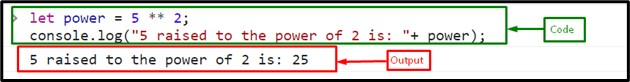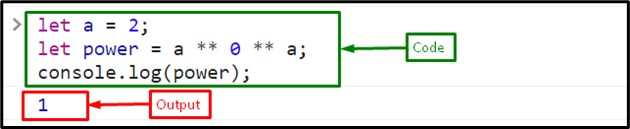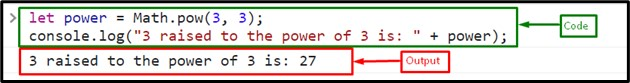# Exponentiation in JavaScript

JavaScript is an adaptable and widely utilized programming language. It offers developers many features along with powerful mathematical operations. Among these operations, exponentiation is important which gives the ability to easily solve challenging problems and carry out difficult mathematical operations. It is commonly used in various computational tasks, such as calculations, algorithms, and scientific simulations.

This blog will describe the way to use exponentiation in JavaScript.

## How to Use Exponentiation in JavaScript?

For using exponentiation in JavaScript, the following approaches are used:

### Method 1: Exponentiation in JavaScript Using ** Operator

The “**” operator is known as the “exponentiation operator” that was added in ECMAScript 2016 (ES7). It is a simple and easy way to find or calculate the exponents of a number in JavaScript. It makes the code simple and easier to read and understand. The operator can be applied to variables and also to the literal values.

#### Example 1: Exponentiation on Literal Values With a Single Exponent

In this example, we will find the exponent of a number using literals. Here, we will find the exponent of “5” which is the 5 raised to the power 2 using the “exponentiation operator” and then, display it on the console:

```let power = 5 ** 2;
console.log("5 raised to the power of 2 is: "+ power);```

As you can see, the output displays “25,” which is the square of “5”:#### Example 2: Exponentiation on Variables With Multiple Exponents

Here, we will find the multiple exponents using the variable and literals. First, we will declare and variable “a” and assign a value “2”:

`let a = 2;`

Now, take multiple exponents, which are “a” raised to the power “0” and raised to the power “a” which will be calculated as “a0 = 1a = 1” (20 = 12 = 1). Keep one thing in mind while using multiple exponentiation operators, which is the right-to-left associativity rule. It means that when multiple exponentiation operators are used consecutively, the rightmost operation is performed first:

`let power = a ** 0 ** a;`

Finally, display the output on the console using the “console.log()” method:
console.log(power);

##### Output### Method 2: Exponentiation in JavaScript Using “pow()” Method

Another way to find the exponent in JavaScript is to use the predefined JavaScript “pow()” method of the “Math” class. It accepts two arguments, the “base” and the “exponent”, and gives the result of raising the base to the power of the exponent.

#### Syntax

Follow the given syntax for the pow() method:

`Math.pow(base, exponent)`

#### Example

Here, we will call the pow() method by passing base and exponent as arguments and storing the result in the variable “power”. Then, display the resultant value on the console using the “console.log()” method:

```let power = Math.pow(3, 3);
console.log("3 raised to the power of 3 is: " + power);```

The output prints the square of 3 or the 3 raised to the power 3, which is “27”:That was all about the exponentiation in JavaScript.

## Conclusion

Exponentiation is a fundamental mathematical operation utilized in different programming scenarios. In JavaScript, it can be used with the help of two approaches, including the “exponentiation operator” (**) or the JavaScript predefined “pow()” method. This blog described the way to use exponentiation in JavaScript.